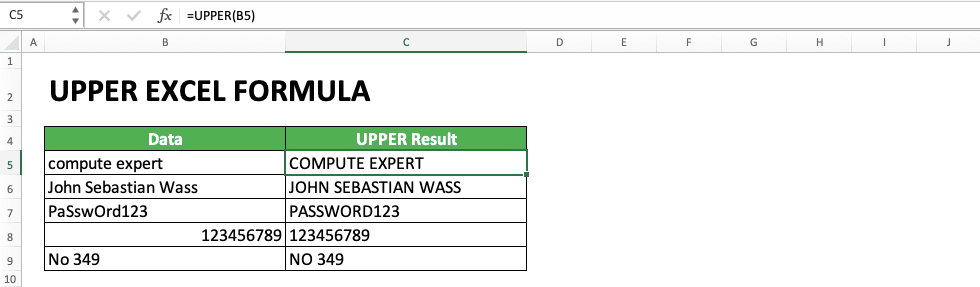How to Use UPPER Excel Formula - Compute Expert

# How to Use UPPER Excel Formula

Home >> Excel Tutorials from Compute Expert >> Excel Formulas List >> How to Use UPPER Excel Formula

In this tutorial, you will learn completely how to use the UPPER excel formula. This formula is useful to convert all letters in the data that becomes its input into uppercase letters.

Disclaimer: This post may contain affiliate links from which we earn commission from qualifying purchases/actions at no additional cost for you. Learn more

## UPPER Usefulness

The UPPER formula is useful to convert all letters in data into uppercase letters.

## UPPER Result

The result you will get from UPPER is data with the letters in it are all uppercase letters.

## Excel Version in Which the UPPER Formula Can Start to Be Used

UPPER can start to be used since excel 2003.

## The Way to Write It and Its Input

The UPPER writing in excel can be illustrated as follows:

=UPPER(text)

As you can see above, the input needed by this formula writing is just one. It is text, the data you want to change all its letters into uppercase letters.

## Example of Usage and Result

The following will give and explain the example of UPPER usage, its writing and the result you get from it.In the example, we can see what is the change made by UPPER to data. UPPER will transform all letters in your data into uppercase letters. It won’t do anything to any other data types (numbers, spaces, etc).

The way to use this formula is pretty easy as you can see in the example. You just need to input the data which letters you want to convert into this formula. Press enter and the letters in the data will all be converted into uppercase letters!

## Writing Steps

The following part will explain the steps to write the UPPER formula in excel. This formula is pretty easy to use because it only needs the data input in which its function will be applied.

1. Type an equal sign ( = ) in the cell where you want to put the result of your UPPER2. Type UPPER (can be with large and small letters) and an open bracket sign after =3. Input the data or cell coordinate where the data you want to convert all the letters is. If you want to type it manually without using a cell coordinate, don’t forget to type the data between two quotes signs (“”)4. Type a close bracket sign5. Press Enter
6. The process is done!## Convert All Letters into Lowercase: LOWER

Want to do the opposite of the UPPER function, which means converting all letters in data into lowercase letters? Use LOWER!

The way to write LOWER is similar to UPPER. The input it needs is the same, which is the data in which you want to convert the letters. Generally, the way to write it can be illustrated as follows:

=LOWER(text)

And here is the example of the LOWER formula usage in excel and the result you get from it.As you can see in the screenshot, LOWER does the opposite of UPPER. It converts all letters in data into lowercase letters. It also doesn’t do anything to data besides letters (numbers, spaces, etc won’t be changed after they are inputted into LOWER).

## Convert the First Letter of Each Word into Uppercase Letters and Other Letters into Lowercase: PROPER

What if we want to convert a text into a title format? That means the first letters of each word in it are converted into uppercase letters and other letters into lowercase letters?

There is also a formula to do that in excel, named PROPER.

The way to write PROPER and its input is similar to UPPER. Generally, here is the illustration of the PROPER writing in excel.

=PROPER(text)

And here is its usage example and the result you get from it.In the example, you can see the PROPER result form and how the PROPER writing and input are similar to UPPER. PROPER will convert the first letter of each word in data into uppercase letters and other letters to lowercase letters. Like UPPER, the data form besides letters isn’t changed after it is inputted to PROPER.

If you want to learn deeper about PROPER, visit this tutorial!

## Exercise

After you have learned how to use UPPER, now you can deepen your understanding about it by doing the exercise below.

### Questions

Do all the following instructions in the gray-colored cell on the appropriate row number!
1. Use UPPER to convert all the letters in the first data on the left cell into uppercase letters! What is the result of the first data?
2. Use UPPER to convert all the letters in the second data on the left cell into uppercase letters! What is the result of the second data?
3. Write your name in small letters form as the input of your UPPER!

If you input a date into UPPER, then you will get its equivalent number in excel.

Related tutorials you should learn:

Get updated excel info from Compute Expert by registering your email. It's free!